# Profit and loss Formulas

Profit and loss problems involve various terms like Cost price, Selling price, Marked price and Discount etc. Basically, it is a difference between selling price and cost price.

Cost price is the price paid to purchase an article or a product or we can say it is a cost incurred in manufacturing an article.

Selling price is the price at which a product is sold.

## 6 Commonly used Profit and loss Formulas :

### 1) Calculation of Profit:

Profit or gain = Selling price(S.P) - Cost price(C.P)
in other ,
⇒ Selling Price = Cost Price + Profit

### 2) Calculation of Loss

Loss = Cost price - Selling price

### 3) Calculation of Gain percentage(%)### 4) Calculation of Loss percentage(%)### 5) Finding relationship between selling price and cost price: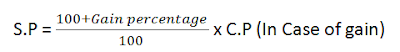For Example: If an article is sold at a gain of 27%, then by using the first formula, you can find that S.P. is 127% of C.P.
Similarly, If an article is sold at loss of 18%, then by using the second formula, you can find that S.P. is 82% of C.P.

### 6) Finding Combined Profit/Loss on sale of Two products

If a person sells two commodities at same prices. On one he gains x% and loses x% on another, then as a whole he will be in loss and the loss percentage will be equal to: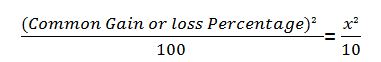Note: Here is an example to find gain in case of dishonesty.
Problem 1: A dishonest dealer professes to sell his goods at cost price but he uses a weigh 960 grammes for 1 kg. How to calculate the gain percentage?
Solution:#### What's trending in BankExamsToday

Smart Prep Kit for Banking Exams by Ramandeep Singh - Download here

##### Join 40,000+ readers and get free notes in your email#### 27 comments:

1.By selling a watch for Rs. 144 a man

loses 10%. At what price should he sell it in

order to gain 10%?

Its in percentage(Profit and loss) notes solved example 2
i got till CP =160rs but then its written Gain required =15% and final answer as Rs 184..plz expailn

1.2.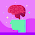SP with CP as Rs.100 = 90 as there is 10 % loss
New SP with CP as Rs.100 = 115 as he needs 15% gain
SP with CP as Rs.100 : actual SP =New SP with CP as Rs.100 : New SP
90 : 144 = 115 : x
90 x = 114 * 115
x = 184

3.90x=144*110
x=15840/90
x=176

2.3.You are correct Vikku.

4.@vikku Ahhh sorry for that, it was 18% instead of 27%. Thanks for correction :)

5.If an article is sold at gain of 27%, then by using first formula , you can find that S.P. is 127% of C.P.
Similarly, If an article is sold at loss of 27%, then by using second formula , you can find that S.P. is 82% of C.P.

in the first case .
gain % -

(100+27) * 100
-------------------- = 127 % Answer
100

and in loss case

(100-27) * 100
------------------ = 73 % loss answer
100

Then why are you showing 82 % in loss case ??

Please correct me

1.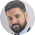Here we are calculating profit or loss on the basis of cost price.

2.hey...! Vikku.... 100-27 or 100-18 once check it

6.A shop keeper sold a hat at 12% profit. Had he sold it for rs.24 more he would have gained 18%, what is the cost price of the hat? plz may i know thw solution,,,

1.SP1= 1.12 CP
SP2-= SP1+ 24= 1.12CP + 24= 1.18CP

Since, 1.12CP + 24 = 1.18CP,
Multiplying both sides by 100, we get-> 112CP + 2400= 118CP
Hence, CP= 2400/6 = 400

Answer is: CP=400.

7.A Shop keeper sold a hat at 12% profit. had he sold it for rs.24 more he would have gained 18%, what is the cost price of the hat?

1.18%-12%= 4%
so 4% of X = 24
x= 400

8.a fruit vendor buys 120 shimla apples at 4 for rs100 and 120 golden apples at 6 for rs100. she decides to mix them and sells at 10 for 200.she will make gain or loss?

1.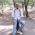9.fruit vendor will get 5 rs of loss

1.how he wl get only 5 rs loss
120 shimla apple means 120/4= 30 sets each of 100= 30*100= 3000 rs shimla apple
similarly 120 golden apple means 120/6= 20 sets each of 100= 2000 rs of golden apple
now toatal appls are 120=120=240 means 240/10= 24 sets & s.p. of one set is 200 means total sp is 200*24= 4800
coast price was 3000+2000=5000 so the loss is 5000-4800=200

10.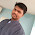A shopkeeppeer purchesed 5char and 7 tables per 5850. On tables he gets 16% profit and on chair 10% profit finally he received 711 as profit find the cost price of tables and chair?

11.18% - 12%= 6 %
6%= 24
1% = 4

100% = 400
i.e CP = 400

12.1.13.sir any shortcuts for Simple &Compound interests??

14.please,,, any1 give me d explaination for d question,,,,, if profit earned after selling an article for 1516 rs is d same as loss incured after selling the article for rs 1492, what is cost prize of the article???????

1.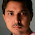profit=sp1-cp(first case)
loss=cp-sp2(second case)
combining both case we get cp=sp1+sp2/2
s0 cp=1516+1492/2=1504

15.By selling a watch for Rs 1440, a man losses 10%. At what price should he sell it to gain 5 %

16.A shopkeeper allows 10% discount on goods when he sells without credit . Cost price of his goods is 80% of his selling price . If he sells his goods by cash then his profit is?

Thanks for commenting. Follow us on Telegram. Search BankExamsToday